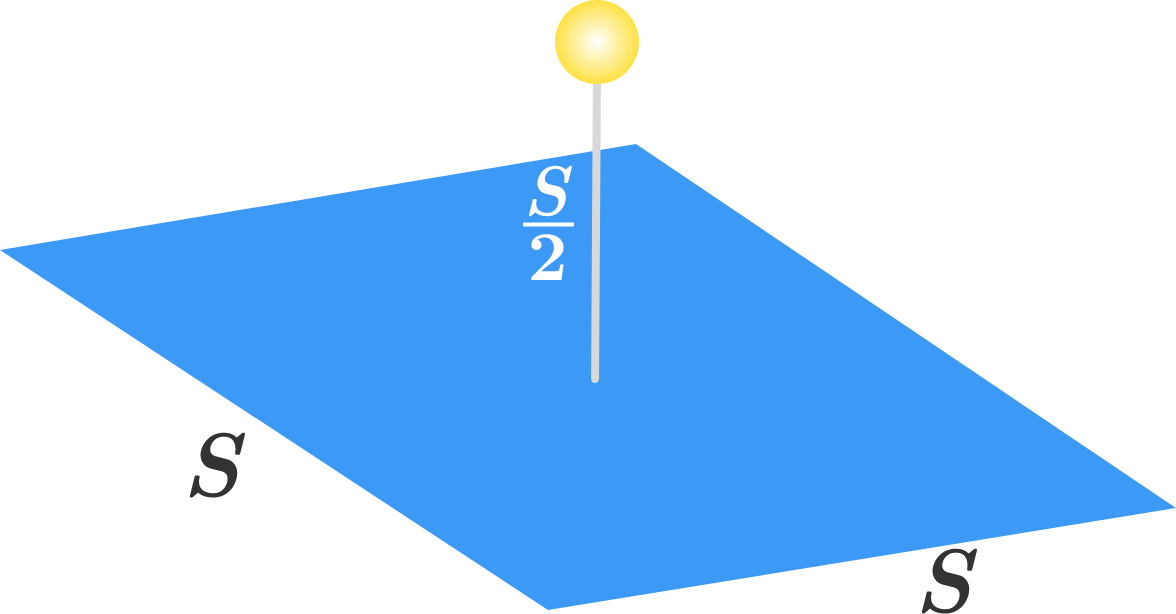In the $xyz$-coordinate system, a thin solid square of side length $S$ lies in the $xy$-plane with its center at the origin. An isotropic light source is positioned at $(x,y,z) = \left(0,0,\frac{S}{2}\right)$.

What percentage of the light energy is incident on the square $($to one decimal place; i.e., $66\frac{2}{3}\%$ would be entered as 66.7$)?$×